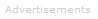# Definition of Partitioning

What is Partitioning?

Partitioning is the distribution of a solute, S, between two immiscible solvents (such as aqueous and organic phases). It is an equilibrium condition that is described by the following equation:

S(aq) ⇄ S(org)

The equilibrium constant for this equilibrium condition is:

K = [S(org)] / [S(aq)]

where S(org) and S(aq) are the solute concentrations in the organic and aqueous phases, respectively, and the equilibrium constant, K, is called the partition or distribution coefficient.

Applications

Partitioning of a solute between two phases is the basis for extractions and chromatographic separations.Search the Dictionary for More Terms